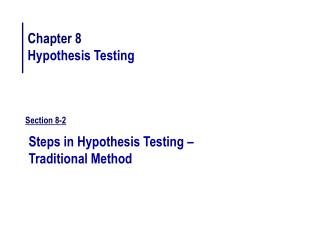DownloadDownload PresentationSection 8-2

# Section 8-2

Télécharger la présentation## Section 8-2

- - - - - - - - - - - - - - - - - - - - - - - - - - - E N D - - - - - - - - - - - - - - - - - - - - - - - - - - -
##### Presentation Transcript

1. Chapter 8 Hypothesis Testing Section 8-2 Steps in Hypothesis Testing – Traditional Method

2. Example 1

3. Section 8-2 Exercise #12b Using the z table find the critical value (or values). Left tail

4. Section 8-2 Exercise #12g Using the z table find the critical value (or values). Right tail

5. Section 8-2 Exercise #12h Using the z table find the critical value (or values). Two - tailed test.

6. Chapter 8 Hypothesis Testing Section 8-3 z Test for a Mean

7. Example 2

8. Section 8-3 Exercise #5 A report in USA TODAY stated that the average age of commercial jets in the United States is 14 years. An executive of a large airline company selects a sample of 36 planes and finds the average age of the planes is 11.8 years. The standard deviation of the sample is 2.7 years. At = 0.01, can it be concluded that the average age of the planes in his Company is less than the national average?

9. Chapter 8 Hypothesis Testing Section 8-3 Exercise #7

10. The average one-year-old (both sexes) is 29 inches tall. A random sample of 30 one-year-olds in a large day care franchise resulted in the following heights. At  = 0.05, can it be concluded that the average height differs from 29 inches?

11. Chapter 8 Hypothesis Testing Section 8-3 Exercise #13

12. To see if young men ages 8 through 17 years spend more or less than the national average of \$24.44 per shopping trip to a local mall, the manager surveyed 33 young men and found the average amount spent per visit was \$22.97. The standard deviation of the sample was \$3.70. At  = 0.02, can it be concluded that the average amount spent at a local mall is not equal to the national average of\$24.44.

13. Chapter 8 Hypothesis Testing Section 8-3 Exercise #17

14. A study found that the average stopping distance of a school bus traveling 50 miles per hour was 264 feet (Snapshot, USA TODAY, March12, 1992). A group of automotive engineers decided to conduct a study of its school buses and found that for 20 buses, the average stopping distance of buses traveling 50 miles per hour was 262.3 feet. The standard deviation of the population was 3 feet. Test the claim that the average stopping distance of the company’s buses is actually less than 264 feet. Find the P-value. On the basis of the P-value, should the null hypothesis be rejected at  = 0.01? Assume that the variable isnormally distributed.

15. Chapter 8 Hypothesis Testing Section 8-4 t Test for a Mean

16. Chapter 8 Hypothesis Testing Section 8-4 Exercise #3a

17. Chapter 8 Hypothesis Testing Section 8-4 Exercise #3b

18. Chapter 8 Hypothesis Testing Section 8-4 Exercise #3c

19. Chapter 8 Hypothesis Testing Section 8-4 Exercise #7

20. The average salary of graduates entering the actuarial field is reported to be \$40,000. To test this, a statistics professor surveys 20 graduates and finds their average salary to be \$43,228 with a standard deviation of \$4,000. Using  = 0.05, has he shown the reported salary incorrect?

21. Chapter 8 Hypothesis Testing Section 8-4 Exercise #9

22. A researcher estimates that the average height of the buildings of 30 or more stories in a large city is at least 700 feet. A random sample of 10 buildings is selected, and the heights in feet are shown: 485 511 841 725 615520 535 635 616 582 At  = 0.025, is there enough evidence to reject the claim?

23. Example 3

24. Chapter 8 Hypothesis Testing Section 8-4 Exercise #13

25. Last year the average cost of making a movie was \$54.8 million. This year, a random sample of 15 recent action movies had an average production cost of \$62.3 million with a variance of \$90.25 million.At the 0.05 level of significance, can it be concluded that it costs more than average to produce an action movie?

26. Chapter 8 Hypothesis Testing Section 8-4 Exercise #17

27. A report by the Gallup Poll stated that on average a woman visits her physician 5.8 times a year. A researcher randomly selected 20 women and the following data was obtained. At  = 0.05 can it be concluded that the average is still 5.8? Use the P - value method.

28. Chapter 8 Hypothesis Testing Section 8-5 z Test for a Proportion

29. Chapter 8 Hypothesis Testing Section 8-5 Exercise #7

30. It has been reported that 40% of the adult population participates in computer hobbies during their leisure time. A random sample of 180 adults found that 65 engaged in computer hobbies. At  = 0.01, is there sufficient evidence to conclude that the proportion differs from 40%?